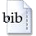User: GuestLogin
Document type:
Zeitschriftenaufsatz
Author(s):
K$\alpha$$\mu$$\alpha$$h̊o$$\gamma$$\iota$$\acute\alpha$$\nu$$\nu$$\eta, M\alpha$$\o̊$$ί$$\alpha$
Title:
Development of hybrid models of teenagers’ travel patterns to school and to after-school activities
Year:
2014BibTeX